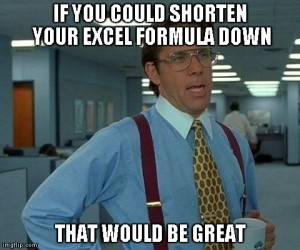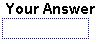# Excel Formula Question #3 from Modeloff 2013 Released with Explanation (3 of 4)

Calling all Excel formula geeks, fans, and enthusiasts out there. It’s that time again! See if you have what it takes to complete the 3rd Excel challenge in this riveting series from the Modeloff 2013 award ceremonies. The 2nd challenge from last week’s post involved using array-entered formulas. Challenge #3 builds off the awesomeness of array-entered formulas. This week’s challenge is probably the easiest one so far, but is hard to optimize since the final solution only contains 22 characters! Big ups to Dan Mayoh, the creator of the challenge for letting us post the challenge and answer. Don’t forget he recently launched a finance consulting company called Fintega Pty Ltd (under construction).## The Excel Formula Challenge

Ground rules are the same as before, create a formula that solves the challenge in the least number of characters without using VBA and all that fancy stuff. Read the first post in this series if you want the full rules. This week, the challenge is only one blue cell. Yup. That’s it. Just one cell to enter your kick ass formula.There is also a large 40X15 block of numbers that you will utilize to solve the challenge:The numbers in this block are a bunch of random integers.

## The Objective

Write a formula that counts how many cells in the number block are less than -10 or greater than +10. Ready, set, gooooo! During the Modeloff 2013 award ceremonies, the contestants easily created formulas to solve this challenge, but the problem was trying to find the shortest formula. I’ll give you a hint, the number of cells that have values less than -10 or greater than +10 is 158.

Give it a shot! Download this file that represents the data discussed above and see if you can create the formula.

I’m going to do things a little differently for this post, and walk you through my thought process for finding the solution before posting the actual solution as per Dan Mayoh. If you simply want to see the 22-character solution and don’t give a crap about my meandering thoughts, then scroll to the bottom and get instantly gratified.

## The Thought Process

As stated earlier, the solution definitely involves the use of array-entered formulas to test all the numbers in the block against the condition greater than +10 or less than -10. It also involves the SUM() function in order to add up the number of cells that meet the condition. Ok let’s get started.

`=SUM(N((E20:S59>10)+(E20:S59<-10)))`

This formula is array-entered (CTRL+SHIFT+ENTER) into cell E17 on the worksheet and it will result in the correct answer of 158.  Why does this work? Well let’s first look at what the two conditions we are testing for: E20:S59>10 and E20:S59<-10. Nothing too crazy here, we are simply checking the entire range of random integers to see which ones are less than -10 and which ones are greater than 10.

The interesting thing about array formulas is that you can put OR() and AND() operators to test your conditions. For array formulas, the syntax to use is the “+” sign to tell Excel that we want to see which numbers are less than -10 OR greater than 10. If we used the “*” sign in between our two conditions, this would tell Excel to look for values that are less than -10 AND greater than 10. This conditional makes no sense because it is impossible for a number to be both greater than 10 and less than -10. Duh.

If we array-enter this formula =SUM((E20:S59>10)+(E20:S59<-10)), we will get the correct result of 158. However, the number of characters in this formula is 32, a whopping 10 more characters than the actual solution. There must be a better way to optimize, so I thought about using the ABS() function which returns the absolute value of whatever number you give it. This would allow me to not compare the range of values to both 10 and -10, but rather just one number.

The next iteration looks like this: =SUM(ABS(E20:S59)>10). I got really excited because this formula only contains 21 characters, which is one less than the correct solution. When I array-entered the formula, the result made me realize I am still just a regular Excel geek compared the top financial modelers out there. The result of this array-entered formula is 0, and I struggled to figure out why. Here’s what I discovered.

## The Discovery

ABS(E20:S59)>10) yields an array of TRUE and FALSE values indicating which values are indeed less than -10 or greater then 10. The array would look something like this (abbreviated to save space):

`{FALSE, TRUE, FALSE, TRUE, TRUE, TRUE, [...]}`

Which is based on the first few values in the number block:

`{9, 22, 0, 15, -14, -18, [...]}`

While we know which values are indeed less than -10 and greater than 10, we cannot simply sum up the resulting array of TRUE and FALSE values. I am not completely sure about this next statement, but the reason why I think  =SUM((E20:S59>10)+(E20:S59<-10)) works is because when you introduce a conditional on top of two other conditions (using the “+” sign), the result is the numeric representation of TRUE and FALSE. You’ll get an array of 0s and 1s which you can easily sum up. If you are only working with one condition, as in the above formula where I used the ABS() function, the resulting array is the non-number values of TRUE and FALSE. If you have a better explanation for why this occurs, please leave a comment below!

Ok, so I know I need to somehow convert these TRUE and FALSE values to numbers. I cheated a little here and Googled for the answer and found a lesser known function called N() which converts non-number values to a number, dates to serials, etc. Exactly what we need! Now the formula looks like this with the N() function doing its job:

`=SUM(N(ABS(E20:S59)>10))`

Array-enter that bad boy and you get 158. However, the character count is at 24 (including the equals “=” sign). Still. Need. To. Optimize. At this point I was getting restless and just wanted to see the solution. I think if I spent another 10 minutes on this sucker I could have come up with the solution.

## The Solution

In all it’s 22 character glory:

`=SUM(N(E20:S59^2>100))`

So simple and elegant. It’s very similar to my solution except the condition is cleverly written to cut down on characters. The E20:S59^2 portion of the formula tells Excel to raise each value to the power of 2 which basically eliminates the need for the ABS() function since any integer raised to the power of 2 will yield a positive number. Of course, we still need our trusty single-character N() function to convert the array of TRUE and FALSE values to 0s and 1s. After you array-enter this guy, you’ll get your solution of 158.

## Conclusion

In the real world, rarely will you encounter the need to optimize your formula to this level of detail. On a project you are usually working under a deadline and whatever formula you come up with that simply works and gets the job done will suffice. There are still two points I want to finish on that this challenge can teach us:

1. Speed up large Excel files – You will work on a file that has thousands or millions of rows, and writing an efficient formula will actually decrease your productivity since Excel will take a long time to think and spit out the answer. This can also lead to your file crashing and data getting lost and you end up playing the whole AutoSave game to recover your file. When you are optimizing a MySQL query, these shortcuts and optimizations can mean the difference between a successful and unsuccessful product.
2. Formula sex factor – There’s no other way to say it. Seeing a really simply formula that can break down a complex problem and spit out the answer is just sexy. I may be taking this too far, but understanding why a formula works allows you to think about other data problems differently. In computer programming parlance, this is like learning a new module and being able to use it over and over again in another part of your program.Whew, that was a lot of talking from me for a 22-character formula. Did you come up with an alternative solution that is less than 22 characters?